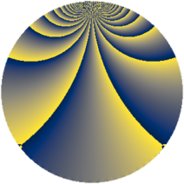# Properties

 Label 3744.1.ehLevel $3744$ Weight $1$ Character orbit 3744.eh Rep. character $\chi_{3744}(883,\cdot)$ Character field $\Q(\zeta_{8})$ Dimension $16$ Newform subspaces $1$ Sturm bound $672$ Trace bound $0$

# Related objects

## Defining parameters

 Level: $$N$$ $$=$$ $$3744 = 2^{5} \cdot 3^{2} \cdot 13$$ Weight: $$k$$ $$=$$ $$1$$ Character orbit: $$[\chi]$$ $$=$$ 3744.eh (of order $$8$$ and degree $$4$$) Character conductor: $$\operatorname{cond}(\chi)$$ $$=$$ $$416$$ Character field: $$\Q(\zeta_{8})$$ Newform subspaces: $$1$$ Sturm bound: $$672$$ Trace bound: $$0$$

## Dimensions

The following table gives the dimensions of various subspaces of $$M_{1}(3744, [\chi])$$.

Total New Old
Modular forms 48 24 24
Cusp forms 16 16 0
Eisenstein series 32 8 24

The following table gives the dimensions of subspaces with specified projective image type.

$$D_n$$ $$A_4$$ $$S_4$$ $$A_5$$
Dimension 16 0 0 0

## Trace form

 $$16q + O(q^{10})$$ $$16q + 16q^{22} - 16q^{55} + O(q^{100})$$

## Decomposition of $$S_{1}^{\mathrm{new}}(3744, [\chi])$$ into newform subspaces

Label Dim. $$A$$ Field Image CM RM Traces $q$-expansion
$$a_2$$ $$a_3$$ $$a_5$$ $$a_7$$
3744.1.eh.a $$16$$ $$1.868$$ $$\Q(\zeta_{32})$$ $$D_{16}$$ $$\Q(\sqrt{-39})$$ None $$0$$ $$0$$ $$0$$ $$0$$ $$q-\zeta_{32}^{3}q^{2}+\zeta_{32}^{6}q^{4}+(-\zeta_{32}-\zeta_{32}^{11}+\cdots)q^{5}+\cdots$$